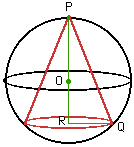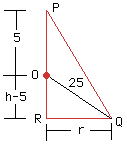Date: Wed, 29 Oct 1997 11:29:13 -0600
Subject: question

1. What real number exceeds its square by the greatest possible amount?

2. The sum of two numbers is k. show that the sum of their squares is at least 1/2 k^2.

3. The sum of two numbers is k. Show that the sum of one number and the square of the other is at least k- 1/4

4. The vertex of a cone and the circular edge of its base lie on a sphere of radius 5 m. Find the dimensions of the cone of maximum volume that can be fitted into the sphere.

5. Find the cone of largest volume that can be inscribed in a sphere of volume V.

thank you
roger h

roger
oac student in Ontario
I AM A STUDENT. I CAN'T ASK MY TEACHERS BECAUSE THEY ARE ON STRIKE FOR "STUDENTS" other then ME.

Hi Roger

Here are a few hints.

1. What real number exceeds its square by the greatest possible amount?

If the number is x then x exceeds its square by x-x^2, so you need to maximize x-x^2.

2. The sum of two numbers is k. show that the sum of their squares is at least 1/2 k^2.

If the numbers are x and y then x+y=k so y=k-x. x^2+y^2=x^2+(k-x)^2 is the sum of their squares. Find the minimum of the this function and show it is 1/2 k^2.

3. The sum of two numbers is k. Show that the sum of one number and the square of the other is at least k- 1/4

4. The vertex of a cone and the circular edge of its base lie on a sphere of radius 5 m. Find the dimensions of the cone of maximum volume that can be fitted into the sphere.Let the center of the sphere be O, the height of the cone be h and the radius of its base be r. Consider the triangle PRQ.Using the theorem of Pythagoras on triangle ORQ you get that (h-5)^2 + r^2 = 25, or r^2 = 25 - (h-5)^2. Since the volume of the cone is V=1/3 Pi r^2 h you can use the expression for r^2 to write V as a function of h alone.

5. Find the cone of largest volume that can be inscribed in a sphere of volume V.
Cheers,
Penny
Go to Math Central